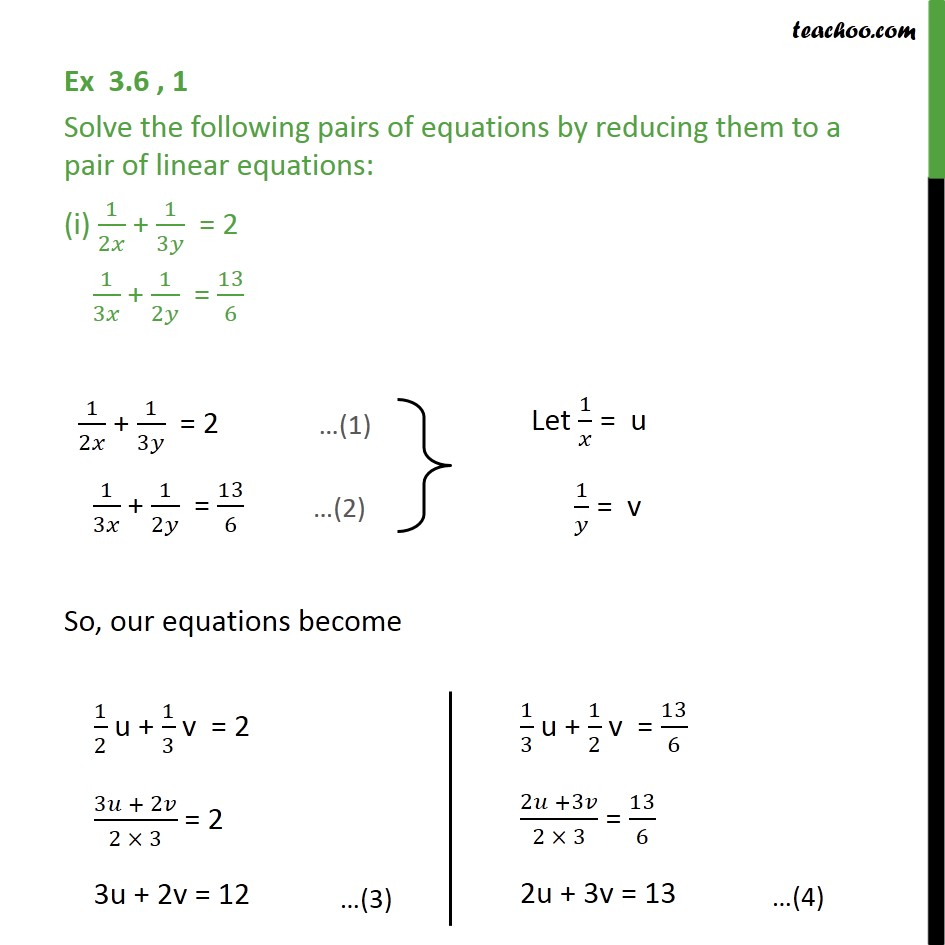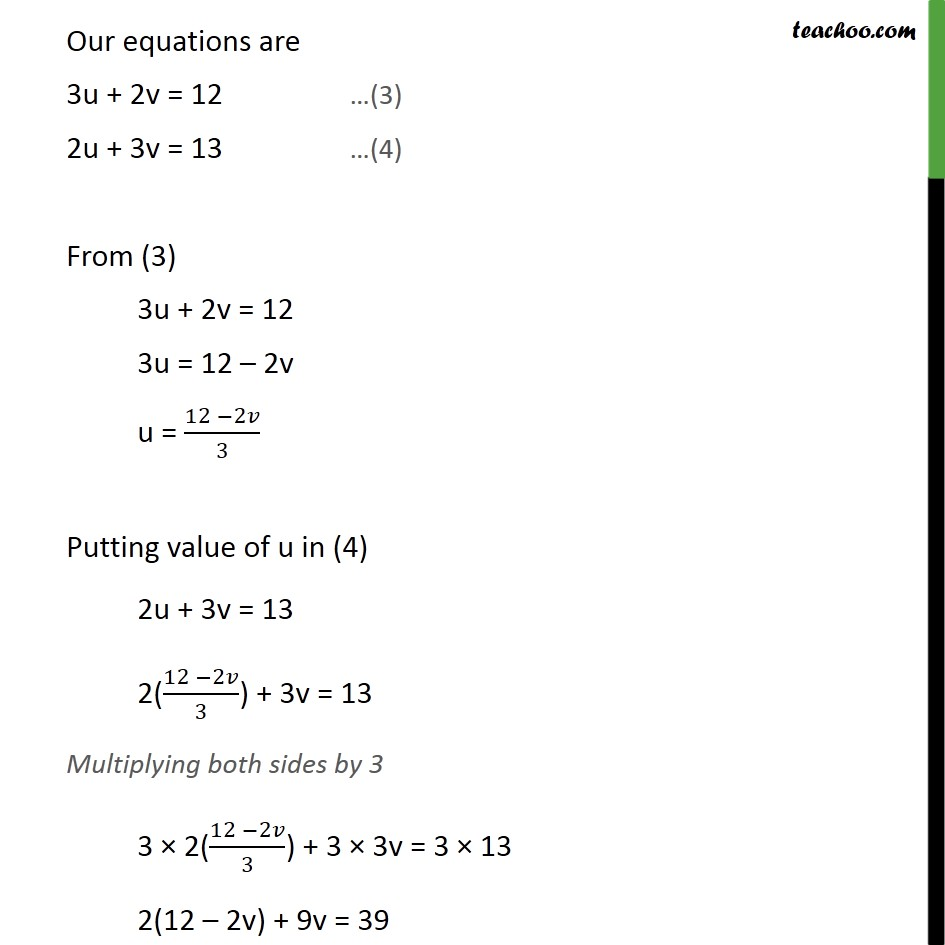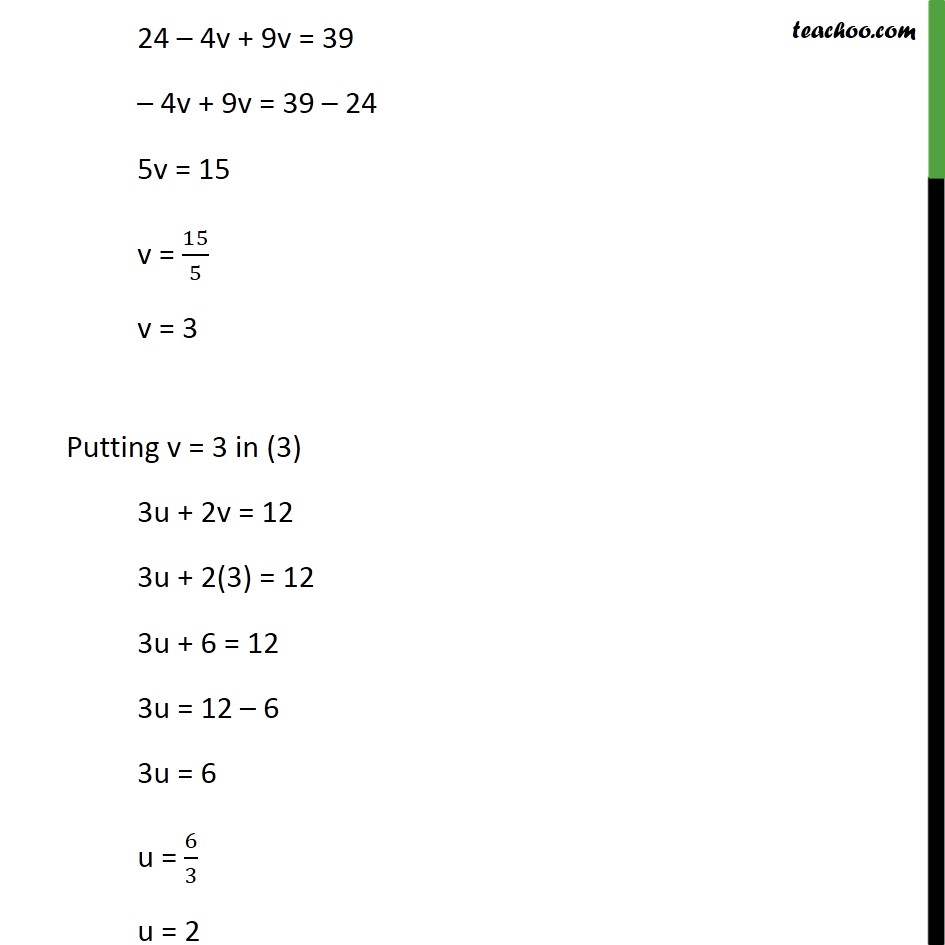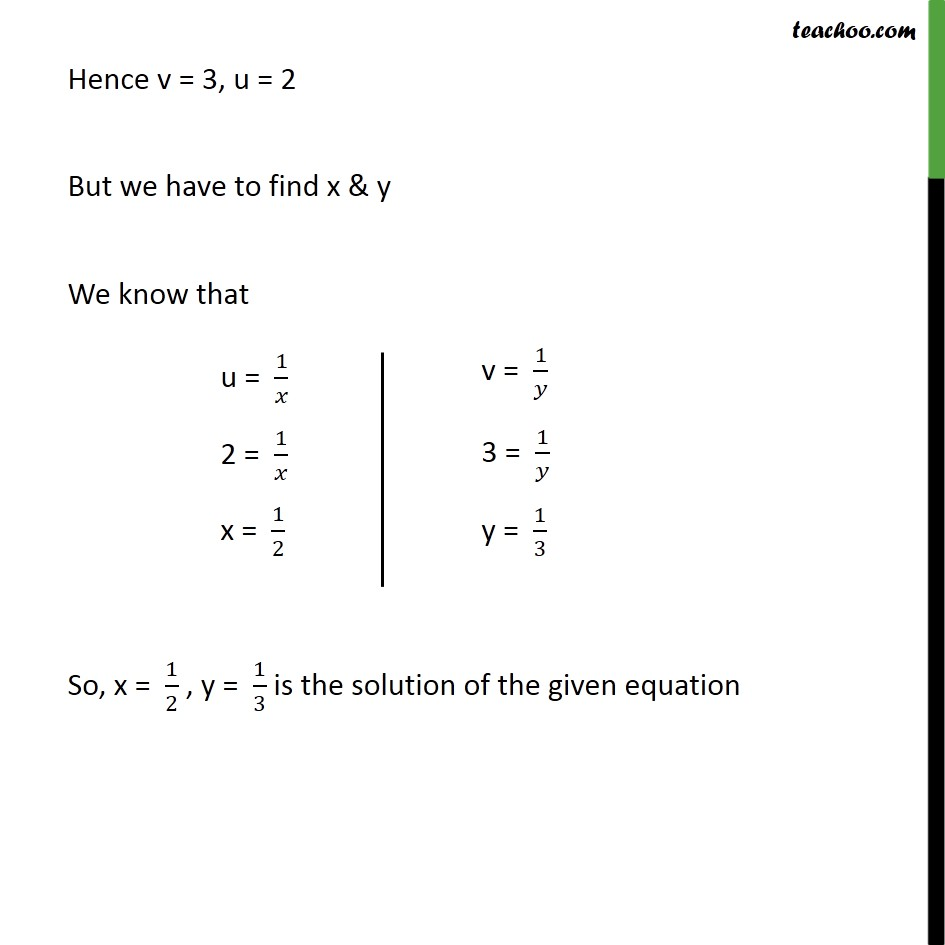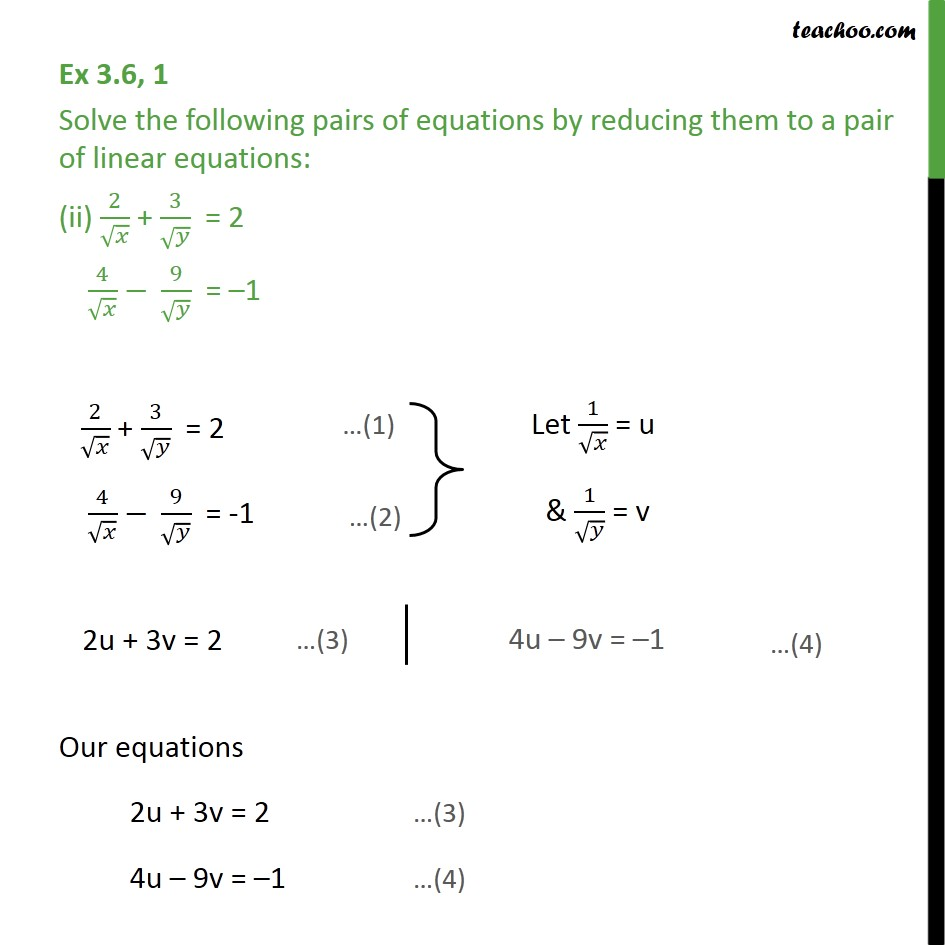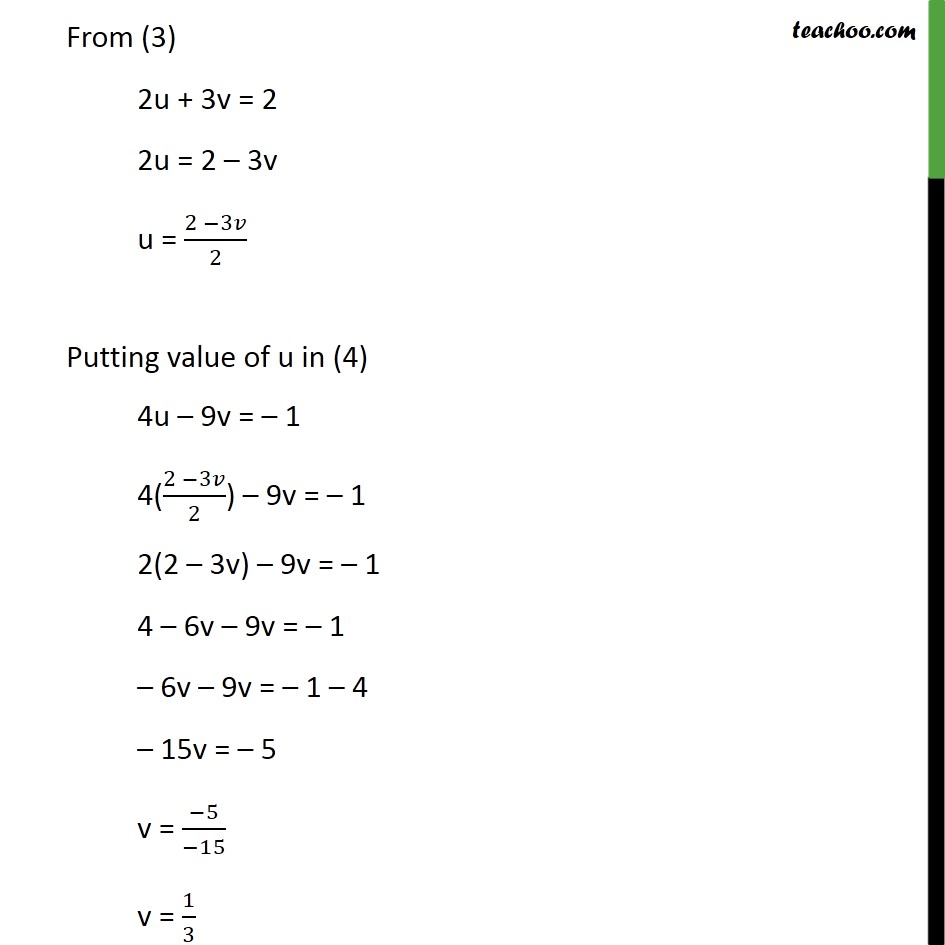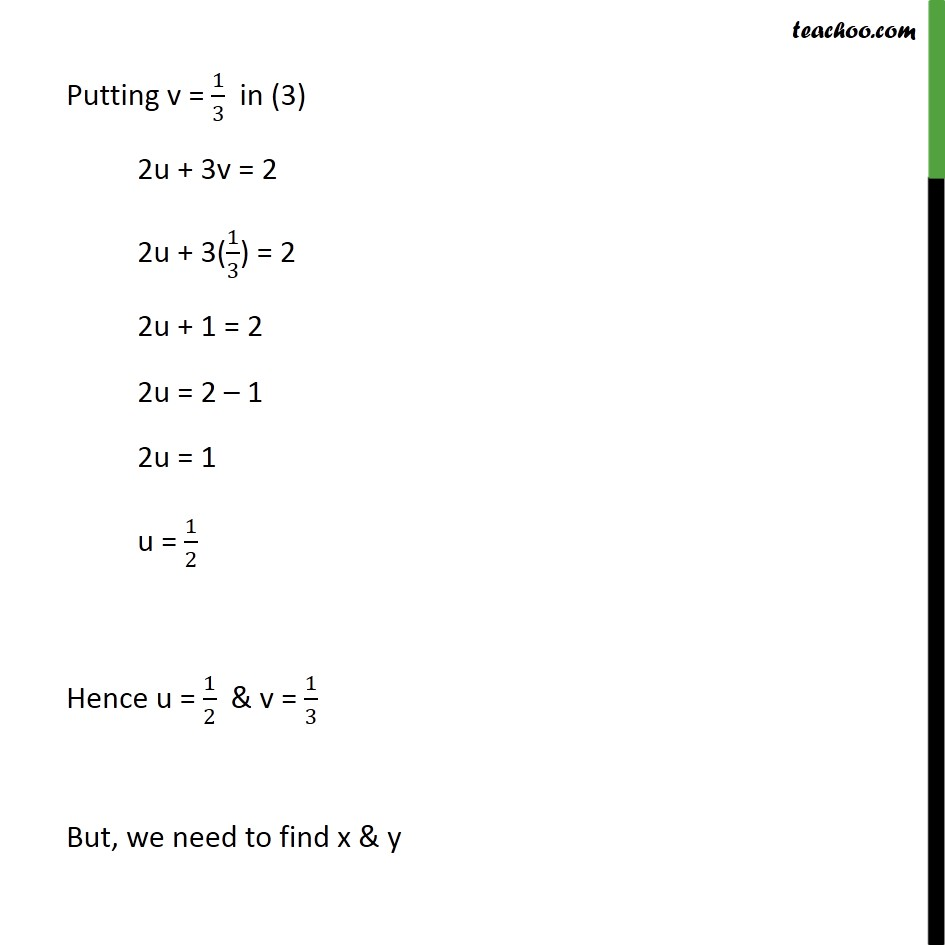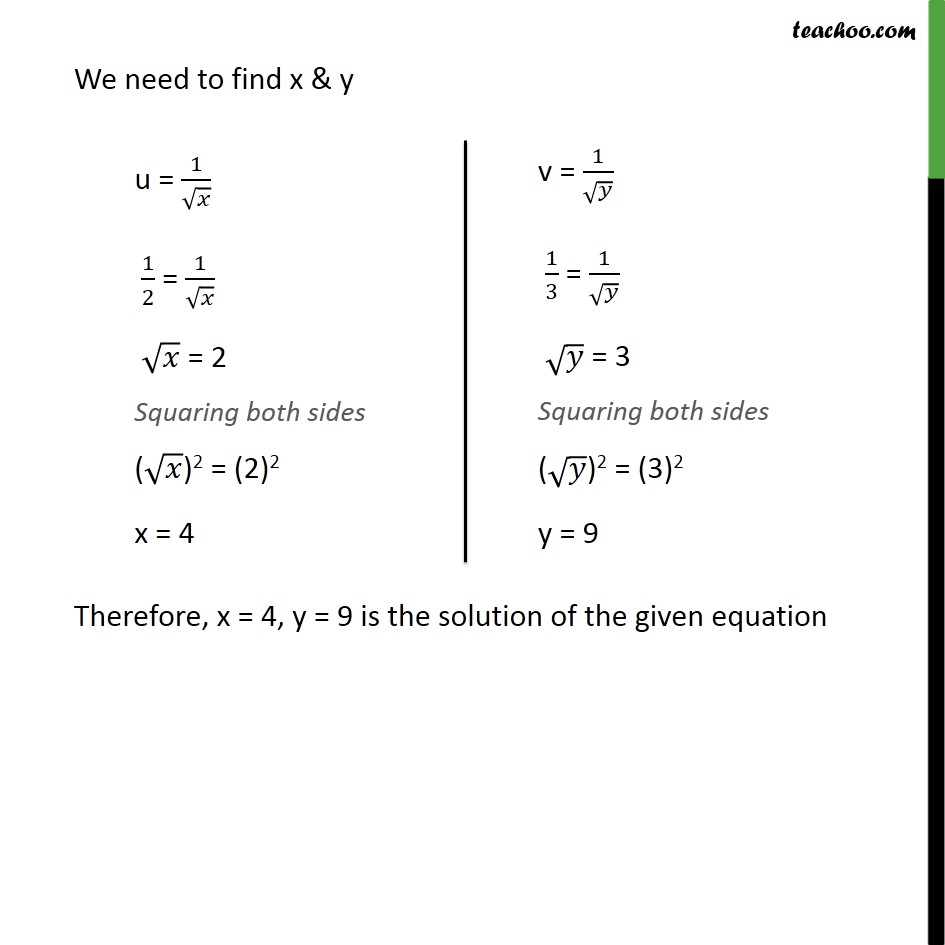1. Class 10
2. Important Questions for Exam - Class 10
3. Chapter 3 Class 10 Pair of Linear Equations in Two Variables

Transcript

Ex 3.6 , 1 Solve the following pairs of equations by reducing them to a pair of linear equations: (i) 1/2𝑥 + 1/3𝑦 = 2 1/3𝑥 + 1/2𝑦 = 13/6 1/2𝑥 + 1/3𝑦 = 2 1/3𝑥 + 1/2𝑦 = 13/6 So, our equations become Our equations are 3u + 2v = 12 …(3) 2u + 3v = 13 …(4) From (3) 3u + 2v = 12 3u = 12 – 2v u = (12 −2𝑣)/3 Putting value of u in (4) 2u + 3v = 13 2((12 −2𝑣)/3) + 3v = 13 Multiplying both sides by 3 3 × 2((12 −2𝑣)/3) + 3 × 3v = 3 × 13 2(12 – 2v) + 9v = 39 24 – 4v + 9v = 39 – 4v + 9v = 39 – 24 5v = 15 v = 15/5 v = 3 Putting v = 3 in (3) 3u + 2v = 12 3u + 2(3) = 12 3u + 6 = 12 3u = 12 – 6 3u = 6 u = 6/3 u = 2 Hence v = 3, u = 2 But we have to find x & y We know that So, x = 1/2 , y = 1/3 is the solution of the given equation Ex 3.6, 1 Solve the following pairs of equations by reducing them to a pair of linear equations: (ii) 2/√𝑥 + 3/√𝑦 = 2 4/√𝑥 − 9/√𝑦 = –1 2/√𝑥 + 3/√𝑦 = 2 4/√𝑥 − 9/√𝑦 = -1 Our equations 2u + 3v = 2 …(3) 4u – 9v = –1 …(4) From (3) 2u + 3v = 2 2u = 2 – 3v u = (2 −3𝑣)/2 Putting value of u in (4) 4u – 9v = – 1 4((2 −3𝑣)/2) – 9v = – 1 2(2 – 3v) – 9v = – 1 4 – 6v – 9v = – 1 – 6v – 9v = – 1 – 4 – 15v = – 5 v = (−5)/(−15) v = 1/3 Putting v = 1/3 in (3) 2u + 3v = 2 2u + 3(1/3) = 2 2u + 1 = 2 2u = 2 – 1 2u = 1 u = 1/2 Hence u = 1/2 & v = 1/3 But, we need to find x & y We need to find x & y Therefore, x = 4, y = 9 is the solution of the given equation

Chapter 3 Class 10 Pair of Linear Equations in Two Variables

Class 10
Important Questions for Exam - Class 10